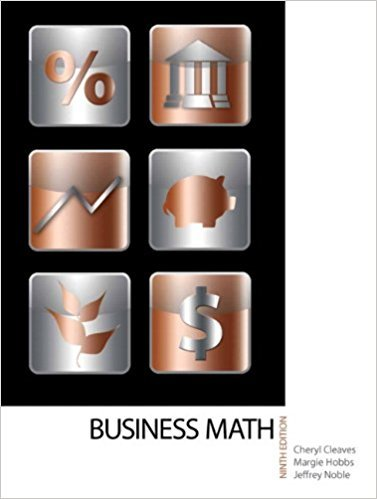×
×

# Solutions for Chapter 13: COMPOUND INTEREST, FUTURE VALUE, AND PRESENT VALUE## Full solutions for Business Math, | 9th Edition

ISBN: 9780135108178Solutions for Chapter 13: COMPOUND INTEREST, FUTURE VALUE, AND PRESENT VALUE

Solutions for Chapter 13
4 5 0 247 Reviews
10
0
##### ISBN: 9780135108178

This textbook survival guide was created for the textbook: Business Math, , edition: 9. Chapter 13: COMPOUND INTEREST, FUTURE VALUE, AND PRESENT VALUE includes 24 full step-by-step solutions. Business Math, was written by and is associated to the ISBN: 9780135108178. Since 24 problems in chapter 13: COMPOUND INTEREST, FUTURE VALUE, AND PRESENT VALUE have been answered, more than 17990 students have viewed full step-by-step solutions from this chapter. This expansive textbook survival guide covers the following chapters and their solutions.

Key Math Terms and definitions covered in this textbook
• Adjacency matrix of a graph.

Square matrix with aij = 1 when there is an edge from node i to node j; otherwise aij = O. A = AT when edges go both ways (undirected). Adjacency matrix of a graph. Square matrix with aij = 1 when there is an edge from node i to node j; otherwise aij = O. A = AT when edges go both ways (undirected).

• Circulant matrix C.

Constant diagonals wrap around as in cyclic shift S. Every circulant is Col + CIS + ... + Cn_lSn - l . Cx = convolution c * x. Eigenvectors in F.

• Column space C (A) =

space of all combinations of the columns of A.

• Condition number

cond(A) = c(A) = IIAIlIIA-III = amaxlamin. In Ax = b, the relative change Ilox III Ilx II is less than cond(A) times the relative change Ilob III lib II· Condition numbers measure the sensitivity of the output to change in the input.

• Cramer's Rule for Ax = b.

B j has b replacing column j of A; x j = det B j I det A

• Cross product u xv in R3:

Vector perpendicular to u and v, length Ilullllvlll sin el = area of parallelogram, u x v = "determinant" of [i j k; UI U2 U3; VI V2 V3].

• Diagonalization

A = S-1 AS. A = eigenvalue matrix and S = eigenvector matrix of A. A must have n independent eigenvectors to make S invertible. All Ak = SA k S-I.

• Hypercube matrix pl.

Row n + 1 counts corners, edges, faces, ... of a cube in Rn.

• Linear combination cv + d w or L C jV j.

• Outer product uv T

= column times row = rank one matrix.

• Positive definite matrix A.

Symmetric matrix with positive eigenvalues and positive pivots. Definition: x T Ax > 0 unless x = O. Then A = LDLT with diag(D» O.

• Random matrix rand(n) or randn(n).

MATLAB creates a matrix with random entries, uniformly distributed on [0 1] for rand and standard normal distribution for randn.

• Schur complement S, D - C A -} B.

Appears in block elimination on [~ g ].

• Schwarz inequality

Iv·wl < IIvll IIwll.Then IvTAwl2 < (vT Av)(wT Aw) for pos def A.

• Standard basis for Rn.

Columns of n by n identity matrix (written i ,j ,k in R3).

• Subspace S of V.

Any vector space inside V, including V and Z = {zero vector only}.

• Sum V + W of subs paces.

Space of all (v in V) + (w in W). Direct sum: V n W = to}.

• Trace of A

= sum of diagonal entries = sum of eigenvalues of A. Tr AB = Tr BA.

• Vector v in Rn.

Sequence of n real numbers v = (VI, ... , Vn) = point in Rn.

• Wavelets Wjk(t).

Stretch and shift the time axis to create Wjk(t) = woo(2j t - k).

×